MATH EXPLORERS' CLUB Cornell Department of Mathematics

# Puzzles and Paradoxes: Infinity in Finite TermsMotivation Tools Application Extension Difficulties References## Extension: Infinite Processes and Structures

We've established that, in at least some circumstances, it's possible to accomplish infinitely many tasks in a finite amount of time. More specifically, if the infinite sequence of tasks is such that the time it takes to do each one forms a geometric sequence with r < 1, then the sum is finite and therefore all the tasks are accomplished in finite time.

Certainly not all sequences of tasks have this property! For example, suppose I am being punished severely for some schoolyard misdeed, and my teacher decides to assign me infinitely many lines to write. If it takes me one minute to write each line, then (viewing each line as a separate task) the corresponding sequence of times is just (1, 1, 1, ...). This is a geometric sequence, sure, but one in which r = 1, so the sum of all the numbers in the sequence can't be found using the formula we derived. In fact, it's easy to see that if each line takes me one minute then I'll never finish writing!

On the other hand, if I could somehow write the first line in 1 minute, the second line in 30 seconds, the third line in 15 seconds, and so on, halving my time with each line, it's not hard to calculate that I'd finish all infinitely many lines in just 2 minutes!

The general idea of doing infinitely many tasks is really not as trite as it might at first seem. So far we've only seen it applied in two cases, one rather esoteric and the other rather silly. But it can actually serve as a very useful conceptual tool.

In his classic work, Gödel, Escher, Bach: An Eternal Golden Braid, Douglas Hofstadter depicts a scene in which Achilles, while conversing with a certain Genie, questions it as to just who (or what) GOD is (pages 103 to 126). The Genie, having made numerous references to this GOD entity already, is all too pleased to answer. He informs Achilles that "GOD" is an acronym which stands for "GOD Over Djinn" (where "Djinn" designates a rather large collection of genies).

Achilles is perplexed and a bit disturbed by this answer. How can an acronym stand for something that includes the original acronym? Isn't that circular? Self-reference? Nonsense?

But the Genie is unperturbed. It calmly explains (though it thought it was well-known) that "GOD" is a recursive acronym; it stands for "GOD Over Djinn", as already noted, and this in turn stands for "GOD Over Djinn, Over Djinn". This second expansion, of course, can be expanded a third time into "GOD Over Djinn, Over Djinn, Over Djinn", and so on. So, unlike regular acronyms (which have just one measly expansion), the recursive acronym "GOD" has an unending sequence of expansions!

Something still seems a bit fishy about recursive acronyms, however. You might object along the following lines: "The real meaning of an acronym comes from what it stands for, so if you can't ever 'get to the bottom' of the expansions, then you can't ever get to the real meaning of the acronym."

Here's a possible response: "Ah, but you can 'get to the bottom' of the expansions! All you need to do is make the expansions one by one, each time twice as fast as the previous expansion. Similarly to the cases we've seen, if the first expansion takes 1 second, the second expansion takes half a second, and so on, you'll have expanded the whole acronym in just 2 seconds!"

In fact, this line of reasoning hits a subtle snag which we'll investigate in the next section when we discuss infinity machines. Nevertheless, I maintain that the idea of a recursive acronym is sensible one, and to convince you of this I'm going to switch to an analogous but somewhat more tangible example of the sort of infinite recursion we encounter in "GOD".

Consider Figure 1: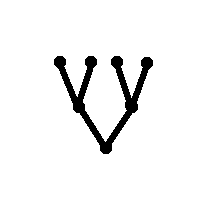Figure 1: The binary tree with three levels

This is an example of a type of structure that is often studied in mathematics: trees. The points, called nodes, are connected to each other in a hierarchical structure by the lines, called edges. In this particular example, our tree has one root node, the bottom-most point, and we can see that from the root node two edges branch upward, leading to the next two nodes. This binary branching at the root node recurs at the next level up, leading to a total of 4 nodes on the third level, at which point the tree terminates.

This recurrent pattern in the tree gives an idea for an alternative way to represent the structure. We might define a new symbol, open-circle, to be given by the rewrite rule shown in Figure 2.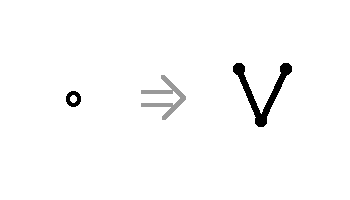Figure 2: The rewrite rule for open-circle

In other words, whenever there is an open-circle in a given diagram, it should be expanded by replacing it with the two-level binary tree.

With this definition in mind, consider the structure depicted in Figure 3.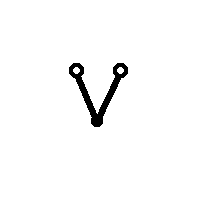Figure 3: A structure presented with open-circle used as a shorthand

Think of the open-circle as analogous to a letter in an acronym: it's a short form for something else. In the same way that understanding an acronym involves expanding all the letters into the words they stand for, understanding the diagram in Figure 3 involves expanding it by replacing the open-circles with what they stand for, as defined in Figure 2. When we do this we see that Figure 3 actually represents the same tree as that shown in Figure 1!

This is neat, but it seems to be a lot of work for not very much gain. Why go through all the trouble of defining open-circle just to use it to present a tree that we could have (and did!) draw explicitly, without a shorthand?

Let us, therefore, try to get a little more out of open-circle. Taking our cue from the contentious "GOD" acronym, let's redefine open-circle according to the rule shown in Figure 4.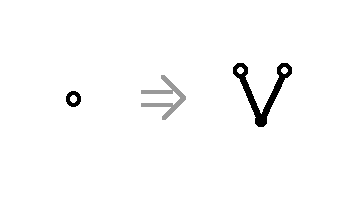Figure 4: The new rewrite rule for open-circle

Self-reference! Whenever we expand an open-circle, we end up with two more open-circles! For example, consider the structure that Figure 3 represents under the new definition of open-circle. Just as when we tried to expand "GOD", we quickly realize that we'll never finish writing and rewriting expansions. The first two such expansions are shown in Figure 5.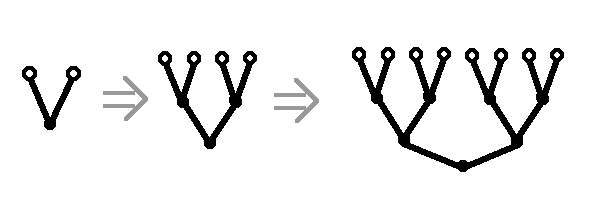Figure 5: The first two expansions according to the rewrite rule in Figure 4

On the other hand, while it was hard to envision what the 'final' result of expanding the "GOD" acronym would be (or even whether such a concept makes sense), in this case there is a much more concrete and natural visualization of what the final result is: with each expansion, the tree becomes one level taller and continues to follow the binary branching pattern. At the 'end', therefore, we should be left with none other than the infinite binary tree -- the tree that looks exatly like all the other k-level binary trees, except that it has no terminating level. It just goes up and up forever, with binary branching all the way.

True, this structure is infinite. But, then again, lots of structures in math are infinite: the natural numbers, the rational numbers, and the real numbers, for example. The set of all points in 3-space is infinite, too. There's nothing in principle wrong with being infinite, so that property can't really count as a strike against the infinite binary tree. Indeed, the infinite binary tree is a widely studied structure in mathematics, and it is very closely related to one of the most popular mathematical structures of all time: the Cantor set.

Exercise 10: Consider yet another rewrite rule for open-circle as given in Figure 6. Using this rule, expand the shorthand structure shown in Figure 7 to figure out why this structure is sometimes called 'the infinite comb'.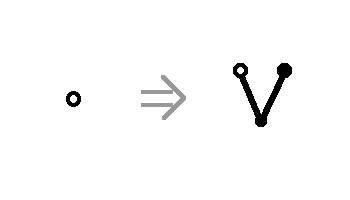Figure 6: Yet another rewrite rule for open-circle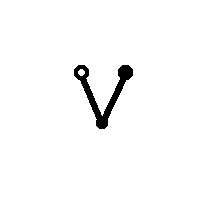Figure 7: The infinite comb?

Still, though, I promised a concrete example wherein infinite recursion makes sense, and what I gave you was an infinitely tall tree -- perhaps not as concrete as you might have hoped. To rectify this, in the remainder of this section we'll take a look at one more structure obtained by a recursive rewrite rule. Unlike the previous two, this structure fits on a single page; indeed, this is perhaps the most surprising thing about it!

Let's begin with a rewrite rule, as shown in Figure 8.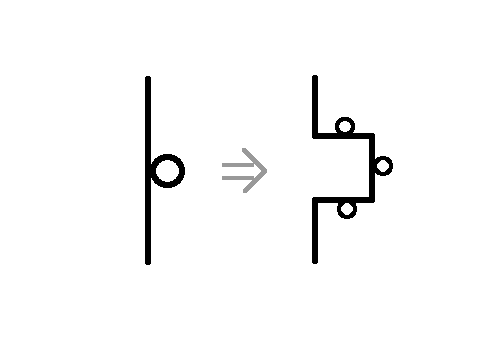Figure 8: The rewrite rule

This rewrite rule says the following: whenever you come across a line (of length L, say) with a little circle touching it as shown on the left of Figure 8, expand it to the shape on the right, where each straight line segment on the right is of length exactly L/3.

With this rewrite rule in mind, consider the structure depicted in Figure 9.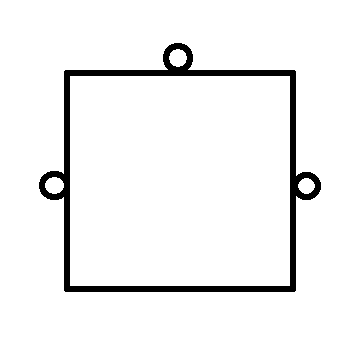Figure 9: The unexpanded structure

Here we have a square, three of whose edges are marked by the circle and so need to be expanded. After one expansion, for example, we obtain the the representation shown in Figure 10.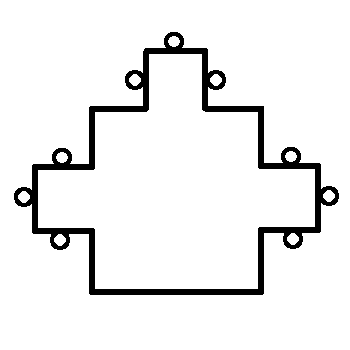Figure 10: After one expansion

Once again, there is an emerging pattern. We begin with a square, and then expand once by 'pushing' a new, smaller square out of each of three sides. If we assume that the original square had side length 1 unit, then the three smaller squares we add to get the first expansion must each have side length 1/3 units. To get the second expansion we have to push three new squares out ofeach of the three squares we obtained in the first expansion, for a total of 9 new squares, each of side length 1/9.

Just as with the binary tree, at the 'end' of this infinite process we'll obtain a figure that has been entirely expanded and therefore has no circle marks at all. Despite the fact that this is an infinite process, based on our knowledge of how the expansions work we can figure out some facts about this 'final' expansion.

First, we can calculate its perimeter by representing it as an (infinite) sum. The original figure (if we ignore the circle marks) has side length 1 and therefore perimeter 4. When we move to the first expansion, we delete the middle third of three of the sides of the original square, which subtracts 1 from the perimeter. Then we add in three new squares (missing one side each) where the middle thirds were deleted. Since each of these new squares has side length 1/3 and three sides, each one adds exactly 1 to the perimeter. Therefore, all in all after the first expansion we've subtracted 1 and added 3 to the perimeter, for a net increase of +2.

A similar argument holds for the second expansion. When we move from the first to the second expansion, we delete the middle third of three sides of each of three squares (namely, the three squares we added in the first expansion). Since each middle third, in this case, has length 1/9, once again we've subtracted a total of 1 unit from the perimeter. Then, at each of these 9 spots of deletion, we add in a new square with three sides of side length 1/9. Each such square thus contributes 1/3 to the perimeter, so altogether they add +3. Again, in the second expansion the perimeter experiences a net total increase of +2.

Exercise 11: Convince yourself that every expansion results in a net total increase of +2 to the perimeter.

The final perimeter, therefore, must be the sum (4 + 2 + 2 + 2 + ...), which of course is not finite. Thus our final figure has infinite perimeter. This shouldn't be too surprising, since we built the shape by an infinite process!

What is surprising is the next result: the final figure has finite area. We can proceed along the same line of reasoning we used to calculate the final perimeter. The original figure is a 1 by 1 square and so has area 1. When we expand the first time, all we're doing is 'pushing out' the boundaries of the original square, adding three new squares each of side length 1/3. Each new square, therefore, has area (1/3)(1/3) = 1/9, so all three together add exactly 1/3 to the area.

At the second expansion we're pushing out 9 new squares, each of side length 1/9. As such, each new square we get in the second expansion has area (1/9)(1/9) = 1/81, and so all nine together add exactly 1/9 to the area.

Exercise 12: Show that at the kth expansion we add exactly 1/(3k) to the area.

The final area, therefore, is just the (infinite, geometric) sum (1 + 1/3 + 1/9 + 1/27 + ...)\$. Lucky for us, we've calculated a formula to deal with infinite geometric sums, especially sums like this one where r = 1/3:

Final Area = S = a/(1 - r) = 1/(1 - 1/3) = 1(2/3) = 3/2.

As claimed, the final structure has a finite area: it's only 1.5 square units! What we've built, then, is a shape with infinite perimeter but finite area -- no small accomplishment!

Exercise 13: I claimed that this figure fits on a single page, but all I proved was that it has finite area. For every positive number A, give an example of a shape with area A that won't fit on a page. Show that the shape we constructed above really does fit on a page.
Exercise 14: Is there a shape with infinite area but finite perimeter?

 Top Next lesson